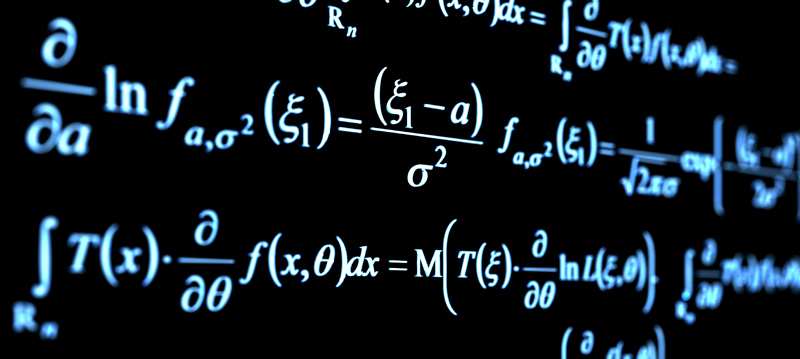# Using Mathematical Principles to Understand the Concept of Fluid Motion in Taijiquan

Sunday, June 26, 2016A lot of Taiji practitioners in their early learning find it extremely difficult to understand or utilize the concept of fluidity of movement. This is especially so when faced with the transition from one movement to the next and how to give the movement finality, while still appearing to be in motion.

From a mathematical perspective, if something is moving at a constant rate we refer to this as a steady velocity. This is what a cruise control on a car provides. The rate of change in distance is constant. At 10km/hour you will move a constant 10Km every hour. In this example the '10' is the constant.

Now suppose that the velocity i s not steady but increasing. Instead of a fixed 10 we are increasing speed at 10km/hour per hour. This is steady acceleration. Without acceleration or its negative, deceleration, you cannot alter your speed. In this case acceleration is 10Km/hour/hour.

In year 10 mathematical terms, speed is the first derivative of distance over time, acceleration the second derivative of distance over time.

It turns out that what makes motion fluid is when you take it one step further and the rate of change of acceleration is itself 'smooth'.

Imagine if you are in one curve and entering a second curve. You are accelerating at 10Km/hour/hour. You have been driving an hour, so you enter at a speed of 10Km/hour. OK, now imagine were instead you were driving at an acceleration of 5Km/hour/hour for two hours. In both cases you hit that corner at the same time and at the same velocity (10Km/hour), however your motion before and after that point is different. If you were to take a video of these two runs and do a video cut/splice at that point you would not see clean motion. This is despite the fact that in both cases you are at the same speed.

The criteria for motion appearing smooth is that the acceleration does not change abruptly. You may have guessed that what we finally want is the rate of change of acceleration to be at least linear. Yes, three levels. If your acceleration is altering at a number of Km/hour/hour/hour it will appear smooth. (In mathematical terms, you want the second derivative to not remain steady and for the 'slope' of the acceleration to see-saw cleanly.)

Taking these mathematical concepts back to the world of Taijiquan, that means you not only have to have the same speed at points of inflection in your moves, but the rates of change must be evenly smooth across the boundary. So, for those of you who are more comfortable with physics and mathematics, hopefully this will resonate and give you something to ponder on while you practice.